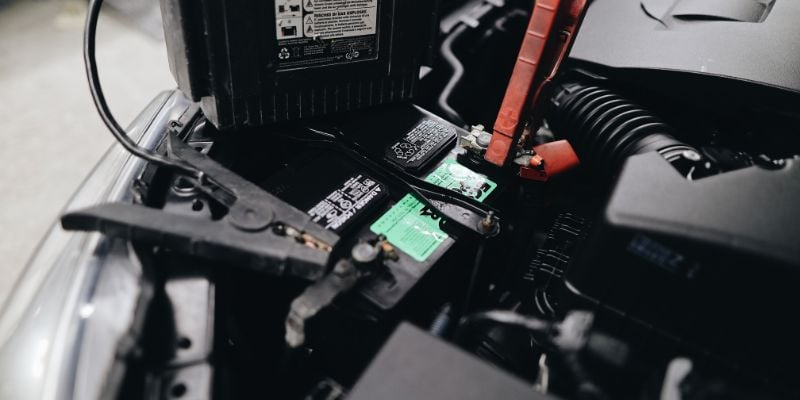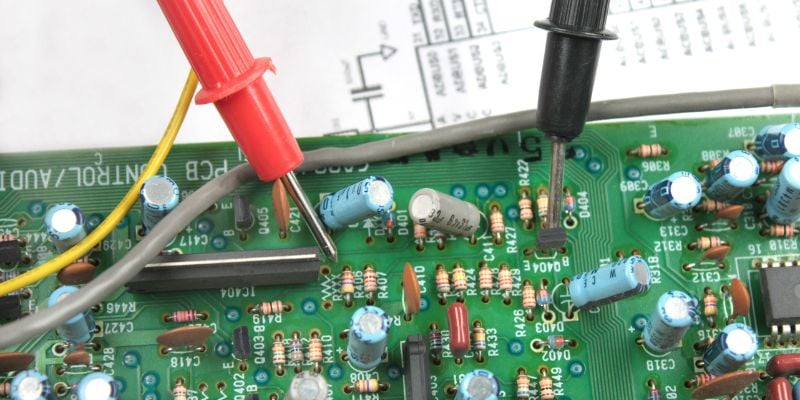Technical Article

# Clarifying Misconceptions About Theoretical vs Physical Circuits

June 15, 2022 by Seth Price

## Control engineering is integrally woven together with electricity and electrical circuits. Even so, sometimes the most basic scenarios become misunderstood through lack of training or experience.

When I started my engineering education, I was an electrical engineering student. After taking several semiconductor fabrication classes, I ended up switching to materials science and engineering.

In my time as an electrical engineering student, there were things I did not understand and was far too afraid to ask. I feel that I am not alone in this, so I will go through a few common misconceptions in resistor networks and circuit analysis that I struggled with as a student. To some of the readers, these will be obvious, but to others, maybe this will answer a few questions or make circuits more clear.

### Battery Circuits and Ohm’s Law

There are literally hundreds of videos on YouTube where someone shorts a car battery with a wrench. The results are similar, with dramatic sprays of sparks, sometimes fire, sometimes the red-hot welding of the wrench to the battery posts or melting the terminals. From Ohm’s Law, it’s easy to see why this happens:

$$I = \frac{V}{R}$$##### Figure 1. Batteries are a common source of energy, but voltage alone does not define the power output capability. Image used courtesy of Canva

A lead-acid car battery is 12 V, and a good steel wrench has very little resistance (assume 0.06 Ohms, for ease of calculation).

$$I = \frac{12~V}{0.0~\Omega}$$
$$I = 200~A$$

In terms of power (heat) generated:

$$P = I^{2} \times R$$

$$P = (200~A)^{2} \times 0.06~\Omega$$

$$P = 2400~W$$

Revisit these calculations with a 9V battery. Plenty of kids learn they can lick the top of a 9 V battery and determine if it still has a charge. A tongue has very low resistance as well (assume 0.03 Ohms, for ease of calculation).

$$I = \frac{9~V}{0.03~\Omega}$$
$$I = 300~A$$

In terms of heat generated:

$$P = I^{2} \times R$$

$$P = (300~A)^{2} \times 0.03~\Omega$$

$$P = 2700~W$$

So the obvious question becomes then: how can a person do this and not become severely injured?

Ohm’s Law does still hold true. The 9 V battery would certainly seek to supply 300 A, but it cannot. The difference lies in the capacity of different batteries to deliver current. A lead-acid or AGM car battery is designed to produce a high cold cranking current of a few hundred (or thousand) amperes to activate the starter solenoid of the car. Car batteries are rated by the number of Cold Cranking Amps (CCA), meaning the amount of current that can be generated from this battery that will flow to the starter solenoid of the engine.

The 9 V battery chemistry simply cannot supply that much current, even if completely shorted. This is why it is safe to lick the top of a battery and not receive a dangerous shock or high current burn (although we still would not recommend attempting this, even for a prank).

### Voltage Drops

When learning about Kirchhoff's Voltage Law, voltage is expressed as voltage “drops”, where there is a certain voltage “lost” as current flows through each device. Also, students are often taught that electricity acts like water flowing through a pipe, where batteries act as the pumps, with each ‘load’ like a water wheel or hydro-powered device reducing the pressure of the water.##### Figure 2. All components - resistors, capacitors, inductors, and semiconductors - they all introduce voltage drops into a circuit. Image used courtesy of Canva

This analogy is a great starting place, but it does not tell the whole story. Imagine a water system where a pump is pumping water through a pipe and through several water wheels. In this system, water wheels are added until the water pressure is not strong enough to turn the last wheel. The water would flow and turn the first wheel and the second and so on, but not the last. The water, lacking pressure, would simply spill over and around the buckets of the final water wheel.

Electricity does not work this way. Unless current can flow all the way through the system, it will not flow at all. For a water system, this does not make sense, as the analogy would be that unless all water wheels turn, none of them will turn.

Back to the voltage drop concept. Suppose there is a series circuit with light bulbs, where lightbulbs are added until finally one does not light. This will never happen; if enough light bulbs are added, the entire strand will go dark; not just one. Current will not flow through some of them and cause them to light, leaving others dark; they are either all light or all dark.

In terms of troubleshooting, the common misconception is that the first dark bulb must indicate the location of the failure. This is not the case, as an open circuit anywhere in the chain, will cause an immediate failure of all the bulbs.

In freshman-level introductory circuit classes, wires are assumed to have no resistance. For theoretical calculations, this is useful; the student can focus on the electrical components rather than worrying about the wires. In the physical world, however, wires do indeed have resistance, and the distance between components matters.

For DC circuits, the resistance is expressed as the resistance per unit length, called resistivity. Not only does the length of the wire matter, but the diameter of the wire is also a factor. There are tables that express the resistivity of different gauge wires. When modeling these wires in a theoretical circuit, they can be modeled as an additional series resistance, where the resistor value is the resistivity per unit length multiplied by the length of the wire in that branch. Where this resistor is located in a branch is of no importance - the current must flow through all components in the branch.##### Figure 3. Control circuits must be carefully designed with proper wire sizes to reduce voltage losses, but also to minimize cost. Image used courtesy of Canva

In the context of system wiring, the appropriate wire gauge, material, and strand count must be carefully matched with the projected current, or the power dissipation of the wire itself will lead to damage and failure of the load.

For AC circuits, the length of the wire is also important. In a basic circuits class, the assumption is at a low enough frequency (or DC) that there is no voltage difference along a wire. The reality is that as frequency increases, there can be what looks like a voltage drop from positions on the same wire. In other words, at one point on the wire, the voltage may be at a positive peak, and another at a negative peak, due to the periodic nature of the signal.

Furthermore, longer wires act as antennas for stray signals. The longer the wire, the bigger the antenna, and the more noise that is possible on the signal. While this may not matter in an introductory class, it can become a major hassle to troubleshoot in sensing loops and other such physical circuits where a detector is looking for a small signal.

### Closing Thoughts